# Angle of Incidence

In optics, the angle of incidence can be defined as the angle between a ray incident on a surface and the line perpendicular to the surface at the point of incidence (called normal). To understand the angle of incidence, we have to first look into the concept of reflection of light. We all know that when a ray of light hits a polished surface like a mirror, it is reflected back.
Table of Content

## Key Pointers

Here are some key points to make you understand the concept of angle of incidence easily.

• The ray of light that hits the polished surface is called the incident ray.
• The ray that gets reflected away is called the reflected ray.
• The point at which the light hits the surface is called the point of incidence.
• If a line is drawn perpendicular to that point, it is called the normal.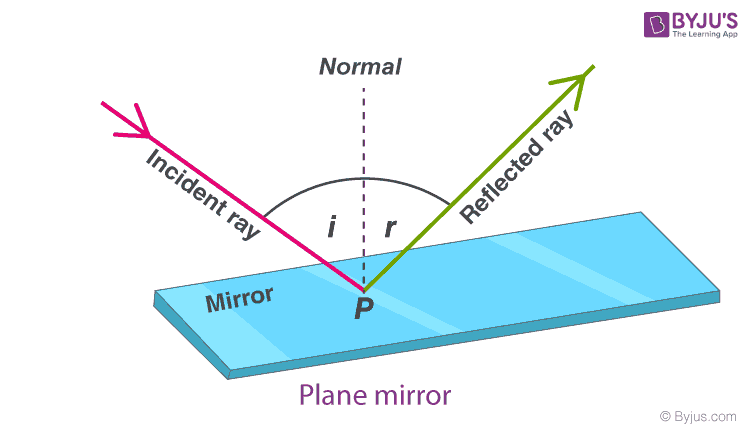The Law Of Reflection

## What is Angle of Incidence?

The incident ray and reflected ray form two angles at the point of incidence:

1. The angle formed between the normal and the incident ray at the point of incidence is called the angle of incidence.
2.  Similarly, the angle formed between the normal and the reflected ray at the point of incidence is called the angle of reflection.

### Angle of Incidence Formula

The angle of incidence is equal to the reflected angle through the law of reflection. The angle of incidence and the angle of reflection is always equal, and they are both on the same plane along with the normal.

### Examples of Angle of Incidence

Example 1:

Consider a light ray which is incident on a flat surface such that it makes an angle of 10° with the surface.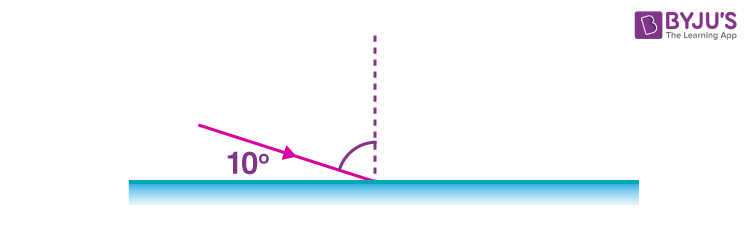1. What is the angle of incidence?
2. What is the angle of reflection?
3. Sketch the diagram for the path of the reflected beam

Solution:

1. We know that the normal to the surface is always 90°. It is given that the light ray is making 10° with the surface. Therefore, the angle of incidence is 90°-10°=80°.
2. From the law of reflection, we know that the angle of incidence is equal to the angle of reflection. Therefore, the angle of reflection is 80°.
3. The below diagram is the sketch for the path of the reflected beam: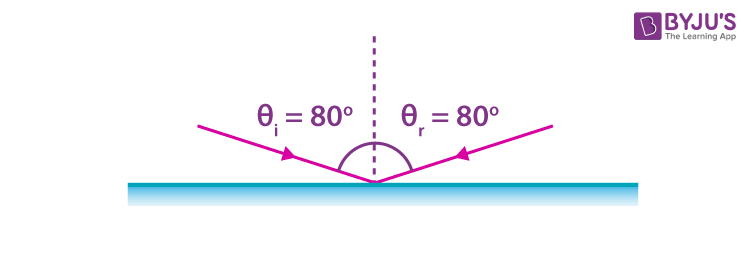Example 2:

The angle at which the light ray strikes the reflective plane surface is 56°. Find

1. The angle of incidence
2. The angle of reflection
3. The angle made by the reflected ray and the surface
4. The angle made by the incident and reflected rays

Solution:

Consider the below diagram to answer the questions: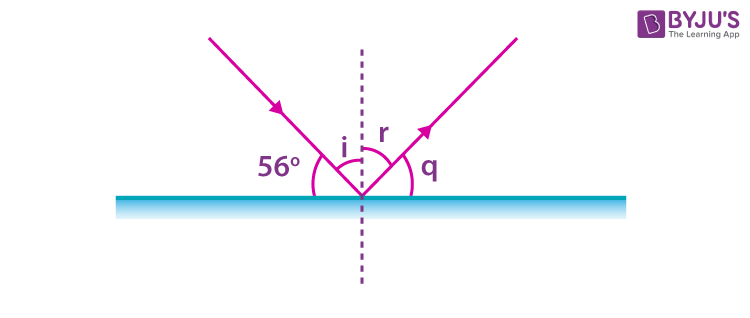1. Angle of incidence = i = 90°-56°=34°
2. From the law of reflection, angle of reflection = r = i = 34°
3. Angle made by the reflected ray and the surface = q = 90°-r = 90°-34°=56°
4. Angle made by the incident and reflected rays = i+r=34°+34°=68°### What is the Relationship between the Angle of Incidence and Angle of Reflection?

When light passes from one medium to another with different densities, its path gets deviated. This phenomenon is called the refraction of light. Like a reflection, there are similar components in refraction too. They are:

• Incident ray
• Refracted ray
• Normal
• Point of incidence

The angle formed at the point of incidence between the incident ray and the normal is called the angle of incidence. The angle formed between the refracted ray and the normal is called the angle of refraction.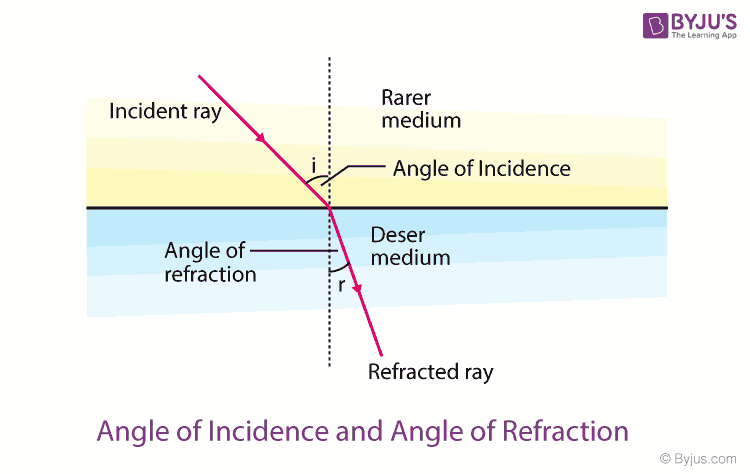Angle of Incidence and Angle of Refraction

The relationship between the angle of incidence and angle of refraction is explained by Snell’s law, which states that the ratio of the sine of the angle of refraction and the sine of the angle of incidence is always constant and equivalent to the ratio of phase velocities of the two mediums it is passing through.

## Frequently Asked Questions – FAQs

Q1

### In total internal reflection, when the angle of incidence is equal to the critical angle, what will be the value of the angle of reflection?

In total internal reflection, when the angle of incidence is equal to the critical angle, the angle of reflection will be 90°.

Q2

### Why is angle of incidence equal to angle of reflection?

The angle of incidence is equal to the angle of reflection because the light ray selects the shortest path to reach the destination. This behaviour of light is known as Fermat’s principle. The same behaviour is shown by the light ray when it gets reflected from the plane surface. Therefore, the angle of incidence and the angle of reflection are equal.

Q3

### What is the angle of reflection if the ray of light is incident normally on a plane mirror?

The ray of light is incident normally on a plane mirror, which means that the angle of incidence is 0° and not 90° degrees. Therefore, the angle of reflection is 0°.

Q4

### What happens to the angle of reflection, if the angle between the incident ray and the mirror is increased?

The angle of reflection decreases when the angle between the incident ray and the mirror is increased. This is because of the glancing angle of incidence. The glancing angle of incidence is the angle formed between the incident ray and the mirror. Also, the sum of glancing angle of incidence and the angle of incidence angle is 90°. So, an increase in the glancing angle results in the decrease of incidence angle. We know that, angle of incidence is equal to angle of reflection. Hence, the angle of reflection decreases.

Q5

### State if the given statement is true or false: Laws of reflection hold good for all types of mirrors.

The given statement is true. That is, the laws of reflection hold good for all types of mirrors.